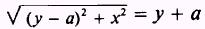Custom SearchTHE PARABOLA A parabola is the locus of all points in a plane equidistant from a fixed point, called the focus, and a fixed line, called the directrix. In the parabola shown in figure 2-8, point V, which lies halfway between the focus and the directrix, is called the vertex of the parabola. In this figure and in many of the parabolas discussed in the first portion of this section, the vertex of the parabola falls at the origin; however, the vertex of the parabola, like the center of the circle, can fall at any point in the plane. The distance from the point (x,y) on the curve to the focus (a,0) isThe distance from the point (x,y) to the directrix x = - a isSince by definition these two distances are equal, we may set them equal: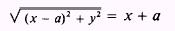Squaring both sides, we have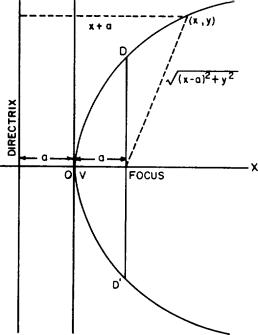Figure 2-8.-The parabola. Expanding, we have x2-2ax+a2+y2=x2+2ax+a2 Canceling and combining terms, we have an equation for the parabola: y2 = 4ax For every positive value of x in the equation of the parabola, we have two values of y. But when x becomes negative, the values of y are imaginary. Thus, the curve must be entirely to the right of the Y axis when the equation is in this form. If the equation is y2 = - 4ax the curve lies entirely to the left of the Y axis. If the form of the equation is x2 = 4ay the curve opens upward and the focus is a point on the Y axis. For every positive value of y, you will have two values of x, and the curve will be entirely above the X axis. When the equation is in the form x2 = - 4ay the curve opens downward, is entirely below the X axis, and has as its focus a point on the negative Y axis. Parabolas that are representative of the four cases given here are shown in figure 2-9. When x is equal to a in the equation y2 = 4ax then y2 = 4a2 and y=2a This value of y is the height of the curve at the focus or the distance from the focus to point D in figure 2-8. The width of the curve at the focus, which is the distance from point D to point D' in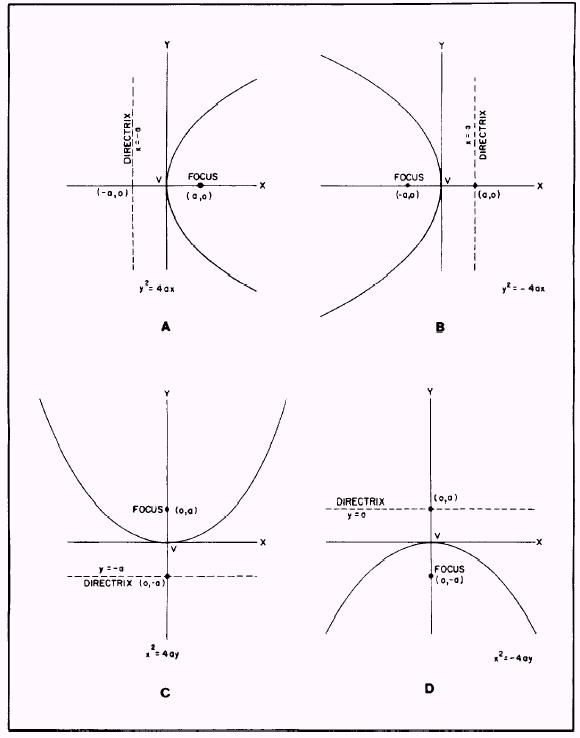Figure 2-9.-Parabolas corresponding to four forms of the equation. the figure, is equal to 4a. This width is called the focal chord. The focal chord is one of the properties of a parabola used in the analysis of a parabola or in the sketching of a parabola. EXAMPLE: Give the length of a; the length of the focal chord; and the equation of the parabola, which is the locus of all points equidistant from the point (3,0) and the line x = - 3. 2-20Figure 2-10.-Sketch of a parabola. SOL UTION: First plot the given information on a coordinate system as shown in figure 2-10, view A. Figure 2-8 shows you that the point (3,0) corresponds to the position of the focus and that the line x = - 3 is the directrix of the parabola. Figure 2-8 also shows you that the length of a is equal to one half the distance from the focus to the directrix or, in this problem, one half the distance from x = - 3 to x = 3. Thus, the length of ais3. The second value required by the problem is the length of the focal chord. As stated previously, the focal chord length is equal to 4a. The length of a was found to be 3, so the length of the focal chord is 12. Figure 2-8 shows that one extremity of the focal chord is a point on the curve 2a or 6 units above the focus, and the other extremity is a second point 2a or 6 units below the focus. Using this information and recalling that the vertex is one-half the distance from the focus to the directrix, plot three more points as shown in figure 2-10, view B. Now a smooth curve through the vertex and the two points that are the extremities of the focal chord provide a sketch of the parabola in this problem. (See fig. 2-10, view C.) To find the equation of the parabola, refer to figure 2-10, view D, and use the procedure used earlier. We know by definition that any point P(x,y) on the parabola is equidistant from the focus and directrix. Thus, we equate these two distances: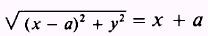However, we have found distance a to be equal to 3, so we substitute: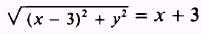We square both sides:Then we expand: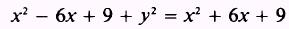We cancel and combine terms to obtain the equation of the parabola:If we check the consistency of our findings, we see that the form of the equation and the sketch agree with figure 2-9, view A. Also, the 12 in the right side of the equation corresponds to the 4a in the standard form, which is correct since we determined that the value of a was 3. Or, since the curve is entirely to the right of the Y axis, then we can apply the formula y2 = 4ax by substituting a = 3 to giveNOTE: When the focus of a parabola lies on the Y axis, the equated distance equation is